@Team 2019-08-13T04:58:57.000000Z 字数 6877 阅读 871

# 《Computer vision》笔记-shufflenet（10）

石文华

## 2、 分组卷积(group convolution)和通道重排（Channel shuffle）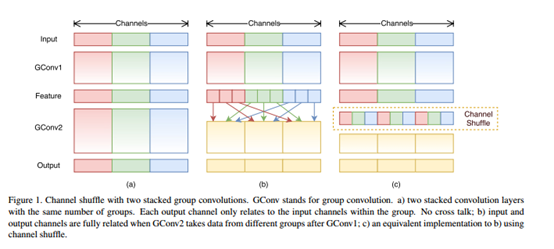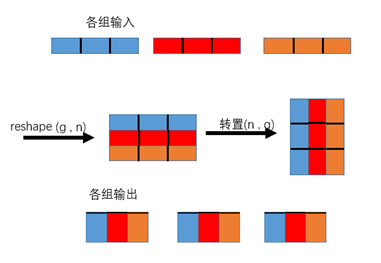## 3、ShuffleNet Units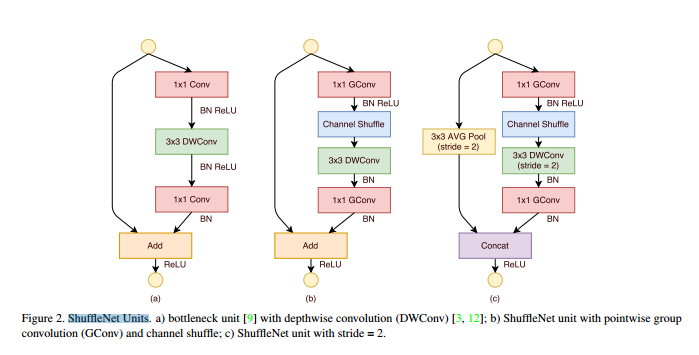## 4、模型结构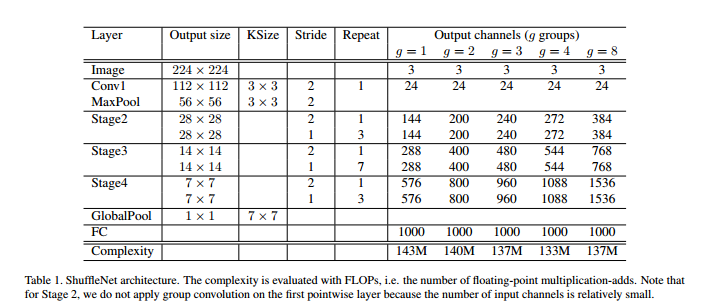## 5、改进（shufflenet v2）

### (1)有效的网络架构设计推导出的一些实用指南：

（Ⅰ）G1:相等的通道宽度可最大限度地降低内存访问成本（MAC）；
（Ⅱ）G2:过多的组卷积会增加MAC；
（Ⅲ）G3:网络碎片降低了并行度；
（Ⅳ）G4:逐元素操作的执行时间是不可忽略的；

（Ⅰ）使用“平衡卷积"（相等的通道宽度）;
（Ⅱ）注意使用组卷积的成本;
（Ⅲ）降低碎片程度;
（Ⅳ）减少逐元素操作。

### (2)ShuffleNet V2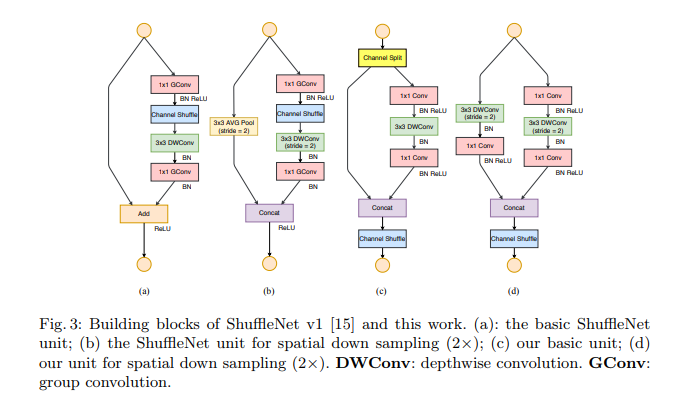## 6、代码：

（1）Conv-Bn-Relu moduule

"""Conv-Bn-Relu moduule"""class ConvBnRelu(nn.Module):    def __init__(self,in_channels,out_channels,kernel_size,stride=1,                 padding=0,dilation=1,groups=1,relu6=False):        super(ConvBnRelu,self).__init__()        self.conv=nn.Conv2d(in_channels, out_channels, kernel_size, stride, padding, dilation, groups, bias=False)        self.bn=nn.BatchNorm2d(out_channels)        self.relu=nn.ReLU6(inplace=True) if relu6 else nn.ReLU(inplace=True)     def forward(self,x):        x=self.conv(x)        x=self.bn(x)        x=self.relu(x)        return x

（2）channel_shuffle

def channel_shuffle(x,groups):    n,c,h,w=x.size()    channels_per_group=c//groups    x = x.view(n, groups, channels_per_group, h, w)    x = torch.transpose(x, 1, 2).contiguous()    x = x.view(n, -1, h, w)    return x

（3）ShuffleNetUtil（V1）

class ShuffleNetUtil(nn.Module):    def __init__(self,in_channels,out_channels,stride,groups,dilation=1):        super(ShuffleNetUtil,self).__init__()        self.stride=stride   #步幅        self.groups=groups   #分组        self.dilation=dilation    #空洞率        assert stride in [1,2,3]           #        inter_channels=out_channels//4     #瓶颈通道的数量        if(stride>1):            self.shortcut = nn.AvgPool2d(3, stride, 1)            out_channels -= in_channels        elif(dilation>1):            out_channels-=in_channels        if in_channels==24:            g=1         else:            g=groups        self.conv1=ConvBnRelu(in_channels,inter_channels,1,groups=g)        self.conv2=ConvBnRelu(inter_channels,inter_channels,3,stride,dilation,dilation,groups)        self.conv3=nn.Sequential(                nn.Conv2d(inter_channels, out_channels, 1, groups=groups, bias=False),                nn.BatchNorm2d(out_channels))    def forward(self,x):        out=self.conv1(x)                      #分组卷积        out=channel_shuffle(out,self.groups)   #channle shuffle        out=self.conv2(out)                    #深度可分卷积        out=self.conv3(out)                    #分组卷积，不进行激活        if self.stride > 1:            x = self.shortcut(x)               #下采样功能的 ShuffleNet unit中，需要将x的分辨率降低，然后才能concat连接，使用concat连接这样做的目的主要是降低计算量与参数大小            out = torch.cat([out, x], dim=1)        elif self.dilation > 1:                #如果采用空洞卷积的话，也是采用concat的连接方式            out = torch.cat([out, x], dim=1)        else:                                   #残差块里面如果没有降低分辨率的，连接方式使用加操作(Add)            out = out + x        out = F.relu(out)        return out

（4）DWConv

class DWConv(nn.Module):    def __init__(self,in_channels,out_channels,kernel_size,stride=1,padding=0, dilation=1,bias=False):        super(DWConv,self).__init__()        self.conv=nn.Conv2d(in_channels,out_channels,kernel_size,stride,                              padding, dilation, groups=in_channels, bias=bias)    def forward(self,x):        return self.conv(x)

（5）ShuffleNetV2Util

class ShuffleNetV2Util(nn.Module):    '''    有效的网络架构设计推导出的一些实用指南：    （Ⅰ）G1:相等的通道宽度可最大限度地降低内存访问成本（MAC）；     （Ⅱ）G2:过多的组卷积会增加MAC；     （Ⅲ）G3:网络碎片降低了并行度；     （Ⅳ）G4:逐元素操作的执行时间是不可忽略的；     基于上述指导原则和研究，有效的网络架构应该：     （Ⅰ）使用“平衡卷积"（相等的通道宽度）;     （Ⅱ）注意使用组卷积的成本;     （Ⅲ）降低碎片程度;     （Ⅳ）减少逐元素操作。    '''    def __init__(self,in_channels,out_channels,stride,dilation=1):        super(ShuffleNetV2Util,self).__init__()        assert stride in [1,2,3]        self.stride = stride        self.dilation = dilation        inter_channels = out_channels // 2   #channel split        if(stride>1 or dilation>1):#带下采样的模块，左边的路径的特征图也需要进行相应的下采样，同时也不使用channel split            self.branch1=nn.Sequential(                    DWConv(in_channels,in_channels,3,stride, dilation, dilation),                    nn.BatchNorm2d(in_channels),                    ConvBnRelu(in_channels,inter_channels,1))        self.branch2=nn.Sequential(#如果带下采样的模块，右侧的路径有所不同，也就是不需要进行channel split                ConvBnRelu(in_channels if (stride > 1) else inter_channels,inter_channels, 1),                DWConv(inter_channels,inter_channels,3,stride,dilation, dilation),                nn.BatchNorm2d(inter_channels),                ConvBnRelu(inter_channels,inter_channels,1)                )    def init_weights(self):        for m in self.modules():            if isinstance(m, nn.Conv2d):                nn.init.kaiming_normal_(m.weight, mode='fan_out')                if m.bias is not None:                    nn.init.zeros_(m.bias)            elif isinstance(m, nn.BatchNorm2d):                nn.init.ones_(m.weight)                nn.init.zeros_(m.bias)            elif isinstance(m, nn.Linear):                nn.init.normal_(m.weight, 0, 0.01)                if m.bias is not None:                    nn.init.zeros_(m.bias)    def forward(self,x):        if(self.stride==1 and self.dilation==1):#如果不进行下采样，则左路不需要做任何运算            x1,x2=x.chunk(2,dim=1)#torch.chunk（input, chunks, dim），与torch.cat()的作用相反。注意，返回值的数量会随chunks的值而发生变化.            out=torch.cat((x1, self.branch2(x2)), dim=1)        else:            out=torch.cat((self.branch1(x), self.branch2(x)),dim=1)        out=channel_shuffle(out,2)#参数2表示groups为2组，因为分成两条路径，生成两组特征图        return out

## 参考：• 私有
• 公开
• 删除